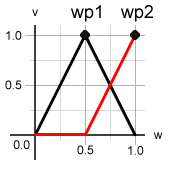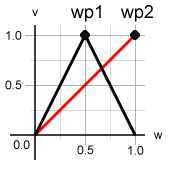# 5.4 WeightPosition Layers

When a weight’s value changes, the WPs are faded in and out, depending on a number of factors. The basic rules are:

• a WP is 0 when the weight is 0

• a WP is 1 when the weight has the value of the WP’s position

• a WP is 0 when the weight has the value of another WP’s position, provided this other WP is visible to the WP in question

The conditional in the final rule allows inbetween dataPoints to behave relative to the main movement the plain dataPoint creates, which is a very useful feature for a similar reason that relative combination dataPoints are handy.

## Layers in Detail

WeightPosition Layers are a means of controlling which WPs can see which. The rule is:

• A WP can “see” all other WPs on the same or lower (i.e. smaller number) layer.

So a WP on layer 1 can see WPs on layers 0 and 1 but not those on layer 2 or higher.

 Hint As a rule of thumb, always have inbetween weightPositions on layer 1 or higher. The layer of the WP at a weight’s value 1 should be 0.

WeightPosition Layers allow you to define a hierarchy between different weightPositions of one weight. DataPoints placed at a wPos on layer 1 or higher are relative to dataPoints placed on a layer 0 wPos.

The following diagrams show how layers change the way in which weightPositions are interpolated. (Note that in this example, the weightPositions have linear interpolation for simplicity’s sake.)

wp1 on layer 0wp1 on layer 1The `w` axis is the value of the weight. The `v` axis is the wPos value. Both images show a setup with two weightPositions. The first (`wp1`) is at the weight’s value 0.5. The `wp2` is at the weight’s value 1. Let’s assume we have placed a dataPoint at each wPos. The `wp2` dataPoint is the plain dataPoint of the weight. As you can see, there are two graphs. The red one is the value of the `wp2` weightPosition and the black shows the `wp1` value.

In the first image, both weightPositions are on layer 0. You can see that both weightPositions are 0 at the weight’s 0 value and 1 at their own position. Their curves are also 0 at the position of any other weightPosition they can see. Since WPs can see all other WPs on the same or (numerically) lower layer, `wp1` sees `wp2` and thus is 0 at the weight value 1. `wp2` on the other hand is 0 at `wp1`.

The second image shows almost the same situation. The difference is that `wp1` is on layer 1. This means that `wp2` cannot see it and behaves as if it was the only wPos of the weight. `wp1` is the same as in the first image, since it can see `wp2`.

## Why do we need this?

There are three reasons for placing a wPos on a higher layer:

1. You might want the inbetween dataPoint at `wp1` to just correct the movement of the plain dataPoint at `wp2`. This can be very useful when the inbetween dataPoint is relative and you change the plain dataPoint. Let’s say you have an inbetween that creates an arced movement when closing the eye. If you now decide to tweak the closed eye, the inbetween will inherit the changes to 50%, so that the movement is still correct.

2. Combination dataPoints that use `wp2` can exist on their own and you don’t need another combination with `wp1`. If `wp1` was on layer 0, a combination with `wp2` would come into play only when the weight reaches values above 0.5. Another combination with `wp1` would be needed. Placing `wp1` on layer 1 will make `wp2` behave as if it was alone, so that combinations with it behave correctly.

3. Certain interpolations only work correctly when used on a sub-layer. E.g. the arc interpolation requires the wPos to be on layer 1 (or higher).